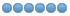# helix.ngc - Where is documentation?

• fc60
• Offline
•25 May 2015 10:33 #58991 by fc60
Greetings,

I was experimenting with the helix.ngc program and would like to know what the following fields are asking for.

#<faceangle> = #12 (=0 face angle)

#<d_angle> = #13 (=0 d angle)

#<spin_notify>= #15 (=0
)

Is there a How-To for each of the NGCGUI programs that are packaged with LinuxCNC?

With kind regards,

Dave

• dgarrett
• Offline
• Platinum Member
•25 May 2015 11:25 #58992 by dgarrett
The ngcgui_lib/ files are provided for teaching by example.
You may have to look at the code or experiment.

For example, the screenshot below shows three helix.ngc invocations
using:
left: x center= 0, d_angle = 0, face angle = 0
middle: x center= 1, d_angle = 90, face angle = 0
right: x center= 2, d_angle = 90, face angle = 45

So the d_angle is the angle of the chord and the face angle
is the rotation angle for the chord.

The spin_notify boolean optionally invokes another subroutine
(utilitysubs/spin.ngc) which by default displays the spindle rpm as a
(debug,) message (lower right). The spin.ngc routine could be
overridden by a like-named routine in [RS274NGC]SUBROUTINE_PATH for other
purposes.
##### Attachments:
The following user(s) said Thank You: fc60